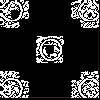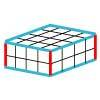#### You may also like### Doplication

We can arrange dots in a similar way to the 5 on a dice and they usually sit quite well into a rectangular shape. How many altogether in this 3 by 5? What happens for other sizes?### Round and Round the Circle

What happens if you join every second point on this circle? How about every third point? Try with different steps and see if you can predict what will happen.### Making Cuboids

Let's say you can only use two different lengths - 2 units and 4 units. Using just these 2 lengths as the edges how many different cuboids can you make?

# Factor-multiple Chains

##### Age 7 to 11Challenge Level

We had quite a few solutions sent in for this challenge. Many just approached the first two questions and those who tried all five sometimes misread - so be careful!  For example the third question says "What numbers cannot appear in any chain?" and many answered by saying what could not start a chain.

First from Chloe and Holly at North Molton:

The solution to Factor Multiple Chains is to find the smallest chain of the factors and multiples is 2, 4, 8, 16 and then the biggest is 5, 25, 50,100.

Then from Haipei at Moorside:

The way to work it out is to find out the pattern linked between the numbers stated to work out the largest and smallest possible combinations.
2, 4, 8 and 16 is the lowest possible combination because 2 is a factor of 4, 8 and 16; and the highest would be 5, 25, 50 and 100 and 5 is a factor of both 25, 50 and 100.

We received three contributions from Grimsargh St Michael.  They were from Klara, Olivia and Isabelle offering 2, 4, 8, 16 as the lowest and either 10, 20, 40, 80 or 7, 21, 42, 84 for the largest.

Karim and Prasidhee from Middleton Primary School sent in their excellently explained solution as a Word document:  AailaandOthers.doc

They also summarised their work:

Question 1: 2, 4, 8,16 We started from the smallest number and kept on multiplying by 2.
Question 2: 12,  24, 48, 96 We experimented with different combinations until we found the correct chain.
Question 3: You times a number by 4 and if it's above 100 the it can't be possible (that's the answer to the question) this is true but leaves out many others.
Question 4: 2-100 they're the smallest and the highest possible number as 2 times 50 = 100
Question 5: 2, 24, 48, 96 Yet again we experimented with the different chains until we found the correct chain.

Jaeyen St Mary and All Saints sent in the following:

What are the smallest blue numbers that will make a complete chain? 2, 4, 8, 16.
What are the largest blue numbers that will make a complete chain? 12, 24, 48, 96.
What numbers cannot appear in any chain? Any odd numbers after 12 can't appear in the chain because you can't divide an odd number by 2 but 3, 5, 7, 9, 11 are allowed, but only to start the chain.I think you may be able to find others as well!
What is the biggest difference possible between two adjacent blue numbers? 48.
What is the largest and the smallest possible range of a complete chain? (The range is the difference between the largest and smallest values.)cBiggest: 12, 24, 48, 96. Smallest: 2, 4, 8, 16.

Mitchell also from St Mary and All Saints sent in this next one and I've added some comments:

What are the smallest blue numbers that will make a complete chain? 2, 4, 8, 16
What are the largest blue numbers that will make a complete chain? 12, 24, 48, 96
What numbers cannot appear in any chain? Any odd numbers after 12 cannot be used because you can't divide odd numbers by 2. This is because an odd number times an odd number equals an odd number. A even number times an even number equals an even number. And an odd number multiplied by an even number is an even number. This is because even beats odd. For example, if you do 11x3 it equals 33. If you do 2x4 it equals 8. And if you do 3x6 it equals 18. In this problem you need to multiply by 2, 4 or 8.This may need further thinking about! Therefore, any odd numbers after 12 can't be used. again I think you may find more.
What is the biggest difference possible between two adjacent blue numbers? 48
What is the largest and the smallest possible range of a complete chain?
(The range is the difference between the largest and smallest values.)

Finally, Aaila and Hamna from Vale View Primary School sent in this extremely systematic solution: AailaandHamna.doc

Well done! You all worked well on this challenge, look out for the next batch of live problems!